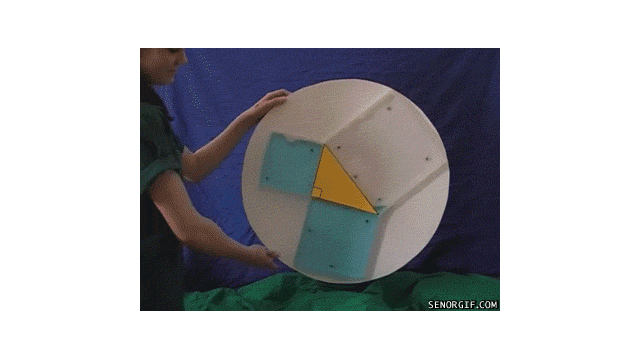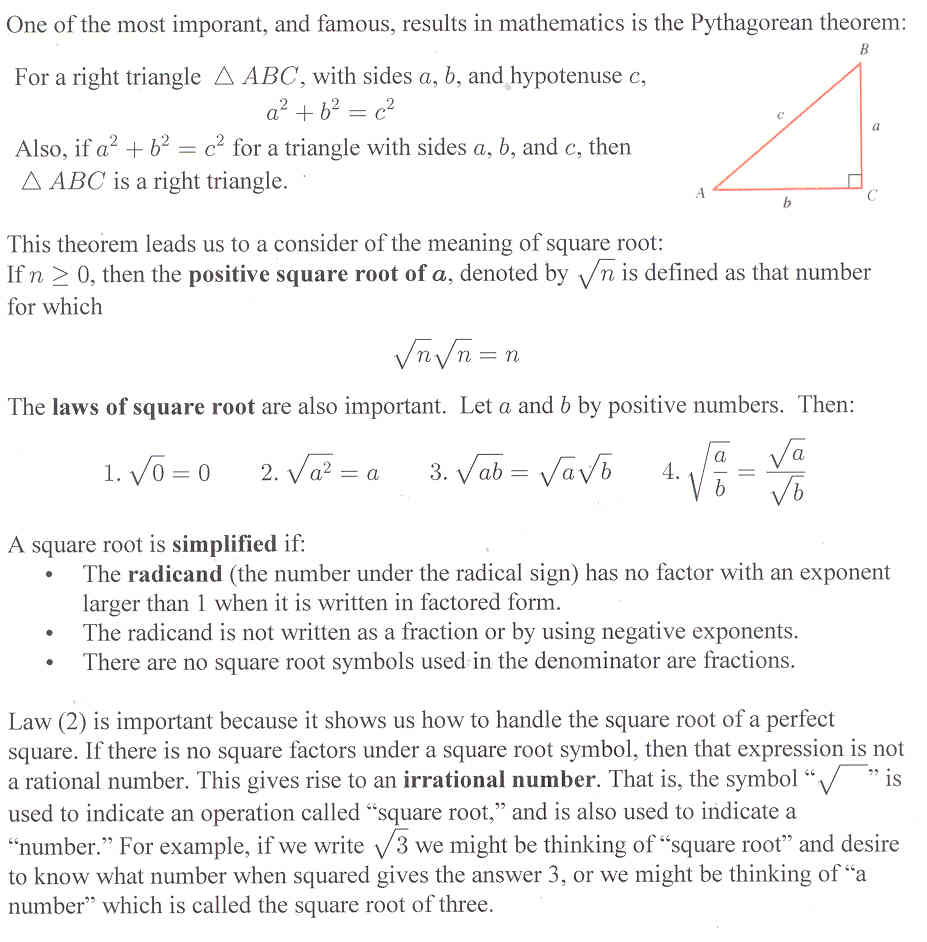# Section 5.5: Irrational Numbers

## 5.5 Outline

1. Pythagorean theorem
1. perfect squares
2. square numbers
3. legs/hypotenuse (There is a nice little graphic showing the meaning of the Pythagorean theorem below)
2. Square roots
1. definition
2. positive square root of n
3. irrational numbers
4. the number pi
3. Operations with square roots
1. simplified square root definition
2. laws of square roots
1. square root of zero
2. square root of a square
3. multiplication of square roots
4. division of square roots## 5.5 Essential Ideas